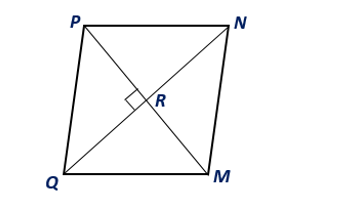Chapter 8.CT, Problem 6CTElementary Geometry For College St...

7th Edition
Alexander + 2 others
ISBN: 9781337614085

Solutions

Chapter
SectionElementary Geometry For College St...

7th Edition
Alexander + 2 others
ISBN: 9781337614085
Textbook Problem

Find the area of rhombus MNPQ given that Q N = 8 ft and P M = 6 ft.To determine

To find:

The area of the rhombus MNPQ.

Explanation

Given:

Rhombus MNPQ given that QN=8 ft and PM=6 ft.

Corollary:

The area A of a rhombus whose diagonals have lengths d1 and d2 is given by A=12d1d2.

Calculation:

From the given, let d1=QN=8 ft., and d2=PM=6 ft

Still sussing out bartleby?

Check out a sample textbook solution.

See a sample solution

The Solution to Your Study Problems

Bartleby provides explanations to thousands of textbook problems written by our experts, many with advanced degrees!

Get Started

In Exercises 1728, use the logarithm identities to obtain the missing quantity.

Finite Mathematics and Applied Calculus (MindTap Course List)

Find the limit. lim0sin+tan

Calculus: Early Transcendentals

Graph the curve x = y 2 sin y.

Single Variable Calculus: Early Transcendentals

Minimize Subject to C=4x+2y+6z x+2y+z42x+y+2z23x+2y+z3x0,y0,z0

Finite Mathematics for the Managerial, Life, and Social Sciences